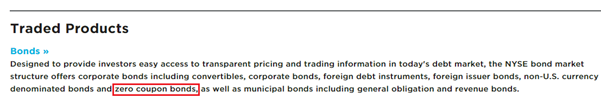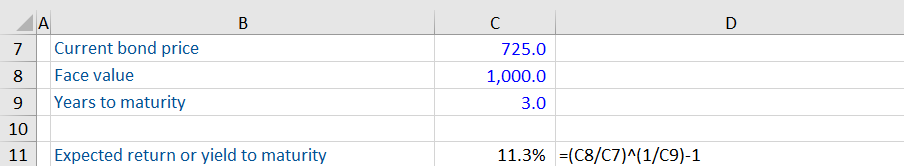## What is a Zero Coupon Bond?

Bonds are debt instruments used by governments and corporations to raise capital. A common feature of most bonds is a regular interest or ‘coupon’ payment. Broadly, there are two types of coupon-paying bonds: 1) fixed coupon bonds where the coupon rate does not change through the life of the bond, and 2) floating coupon bonds where the coupon rate changes in line with a reference interest rate, for example, LIBOR.

Zero coupon bonds are different since they do not pay investors any interest payments between issuance and maturity. Instead, they offer investors a profit upon maturity since the purchase price of a zero coupon bond is always less than the redemption amount received at maturity. Other names for zero coupon bonds are zero bonds, zeroes, and accrual bonds.

## Key Learning Points

• Zero coupon bonds are a type of debt instrument issued by companies and governments to raise capital to fund their operations and growth
• These type of bonds pay no interest (also known as a coupon) to the bondholder
• The holder of a zero coupon bond expects a return upon the bond’s maturity (a higher price than the initial purchase price)
• The yield to maturity (YTM) is the return the bondholder expects to receive and is calculated using the bond’s face value, its current price and the number of years until maturity
• A bond’s maturity or maturity date is the date that the issuer repays the holder the face value of the bond on top of any interest

## Characteristics of Zero Coupon Bonds

### Returns for investors

Zero bonds trade at a discounted price, lower than the amount received at maturity. This difference between the traded price and redemption price is the return realized by investors over the bond’s life. This amount is also known as the accreted interest.

An example is a 10-year zero bond issued at a price of 75%. A bonds price is recorded as a percentage of its face value as opposed to a dollar amount. Investors do not receive any interest over the 10-year term. Upon maturity, the investors will receive 100%.

### Who issues bonds and where are they traded?

Issuers of zero coupon bonds include the US treasury, public corporations, and state and city government entities. Zero coupon bonds are traded on the bond markets of major stock exchanges like the NYSE. Here is a snapshot from the bonds page of the New York Stock Exchange.Bonds typically offer a lower risk compared to the equity market and are easily tradeable in the secondary market.

### Taxes

In many countries, the accreted interest on zero coupon bonds is subject to income taxes. Although investors do not get any returns during the interim period, they need to pay tax on the returns accruing each year. For example, if a bond is offering accreting interest of 50 every year, investors need to pay income tax on this amount, starting from the first year of investment. However, the bonds’ price is adjusted annually to account for this accretion since there is no capital gain tax due upon maturity. If investors sell a zero coupon bond prior to maturity, they must pay tax on capital gains.

## Value and YTM of Zero Coupon Bonds

Bonds are valued by calculating the present value of future cash flows using an appropriate discount rate or interest rate. You can calculate the price of a bond using this formula:

Price of Bond = Face value or maturity value/ (1+interest rate)years to maturity

### Calculating the value of a zero coupon bond

What is the present value of a zero coupon bond with a face value of 1000 maturing in 5 years? The current interest rate is 3%.

Using the formula mentioned above gives 862.6 as the bond’s present value.

### Calculating yield-to-maturity or expected returns

Yield to maturity (YTM) is the expected return on a bond if it is held until maturity. YTM is expressed as an annual rate. In practice, investors are often quoted the price of a bond and would like to calculate the yield that an investment at that price would realize. YTM is calculated by rearranging the above-mentioned formula so that the present value of the future cash flow (the redemption price) equals the current bond price.

(Face value/current bond price) (1/years to maturity)-1

Here is an example of calculating YTM. A zero coupon bond with a par value of 725 is paying 1,000 after 3 years. What is the expected yield to maturity on this bond?= (1,000/750) (1/3)-1

= 11.3%

The bondholder expects a return of 11.3%.